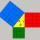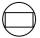Perimeter + square root - examples

1. GardenArea of a square garden is 6/4 of triangle garden with sides 56 m, 35 m, and 35 m. How many meters of fencing need to fence a square garden?
2. RhombusCalculate the perimeter and area of ​​a rhombus whose diagonals are 38 cm and 55 cm long.
3. Square diagonalCalculate length of the square diagonal if the perimeter is 476 cm.
4. StepsHow many steps you save if you go square estate for diagonal (crosswise), rather than circumvent on the two sides of its perimeter with 307 steps.
5. Isosceles right triangleCalculate the area of an isosceles right triangle whose perimeter is 377 cm.
6. ConeCircular cone of height 15 cm and volume 5699 cm3 is at one-third of the height (measured from the bottom) cut by a plane parallel to the base. Calculate the radius and circumference of the circular cut.
7. R TrapeziumRectangular trapezium has bases 12 and 5 and area 84 cm2. What is its perimeter?
8. RhombusFind the length of the other diagonal and area of rhombus. The perimeter of a rhombus is 40 cm and one of the diagonals is of length 10 cm.
9. Triangle SSSCalculate perimeter and area of ​​a triangle ABC, if a=40, b=36 and c=36.
10. Similarity coefficientThe ratio of similarity of two equilateral triangles is 3.5 (ie 7:2). The length of the side of smaller triangle is 2.4 cm. Calculate the perimeter and area of ​​the larger triangle.
11. Rotary coneRotary cone whose height is equal to the circumference of the base, has a volume 229 cm3. Calculate the radius of the base circle and height of the cone.
12. GardenHow many steps of 76 cm circumvent square garden with area 1.8 ha?
13. HeightCalculate height of the equilateral triangle if its perimeter is 8?
14. OPTWhat is the perimeter of a right triangle with the legs 14 cm and 21 cm long?
15. Rectangle and circleThe rectangle ABCD has side lengths a = 40 mm and b = 30 mm and is circumscribed by a circle k. Calculate approximately how many cm is circle long.
16. Triangle eqCalculate accurate to hundredths cm height of an equilateral triangle with a side length 12 cm. Calculate also its perimeter and content area.
17. Square s3Calculate the diagonal of the square, where its area is 0.49 cm square. And also calculate its circumference.
18. The sides 2The sides of a trapezoid are in the ratio 2:5:8:5. The trapezoid’s area is 245. Find the height and the perimeter of the trapezoid.

Do you have an interesting mathematical example that you can't solve it? Enter it, and we can try to solve it.

To this e-mail address, we will reply solution; solved examples are also published here. Please enter e-mail correctly and check whether you don't have a full mailbox.